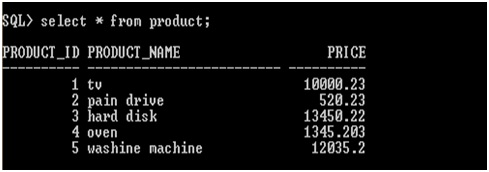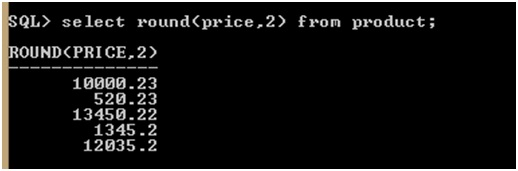# Use of LEN(), ROUND() functions in SQL

In this article, we are going to discuss about the use of LEN(), ROUND() functions in SQL.
Submitted by Bharti Parmar, on January 29, 2019

LEN() and ROUND() are scalar functions which return a single value, based in the input value.

LEN() function is used to find the length of the value in any field.

SYNTAX:

```    SELECT LEN(column_name) FROM table_name;
```

ROUND() function is used for Rounds a numeric field to the number of decimals specified.

TABLE:SYNTAX:

```    SELECT ROUND(column_name,decimals) FROM table_name;
```

Column name and decimal parameter is required to round with specific number of decimal to be returned.

Example:NOTE: As you all know that different database systems ensure passing differently than you might imagine. When rounding a number with a fractional part to an integer, we round any number .1 through .4 DOWN to the next lower integer and .5 through .9 UP to the next higher integer. Many database systems have adopted the IEEE 754 standard for arithmetic operations, so the default rounding behavior is "round half to even." In this scheme, .5 is rounded to the next even integer. So, both 1.5 and 2.5 would be rounded to 2.

Conclusion:

In this article, we have learn how to use LEN(), ROUND() functions in SQL and SQL queries? We will know more about different or use of another scalar function in SQL. Have a great day! Happy Learning!